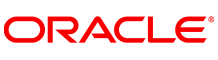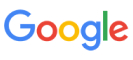New update is available. Click here to update.
Topics

# Matching Queries

Hard0/120
Average time to solve is 45m## Problem statement

You are given an array/list ‘QUERY’ consisting of ‘N’ strings and a string ‘PATTERN’. For each valid integer ‘i’ (0 <= ‘i’ < N), Check whether ‘QUERY[i]’ matches ‘PATTERN’ or not.

‘QUERY[i]’ matches the string ‘PATTERN’, if we can insert some lowercase letters in the ‘PATTERN’ so that it equals the ‘QUERY[i]’.

Your task is to return a boolean array/list of size ‘N’ where the element at index ‘i’ is True, if and only if ‘QUERY[i]’ matches with ‘PATTERN’ otherwise, it is False.

Example :
``````Consider ‘QUERY’ = [“Coding”, “CodiNg”, “COdiNG”, “Ninja”, “CNinja”] and string ‘PATTERN’ = “CN”
Then “CN” equals “CodiNg” by inserting lowercase letters “o”, “d”, “i”, and “g” like this -:  “C” + “odi” + “N” + “g” =  “CodiNg”.
“CN” equals “CNinja” by inserting lowercase letters “i”, “n”  “j” and “a” like this -:  “CN” + “inja” = “CNinja”.
No other string in ‘QUERY’ can match with “CN” by inserting some lowercase letters.
Thus, we should return the boolean array -: [False, True, False, False, True].
``````
Detailed explanation ( Input/output format, Notes, Images )
Constraints :
``````1 <= T <= 50
1 <= N <= 10^3
1 <= |QUERY[i]| <= 10^3
1 <= |PATTERN| <= 10^3
‘PATTERN’ has only lowercase or uppercase English letters.
‘QUERY[i]’ has only lowercase or uppercase English letters.

Where ‘T’ is the total number of test cases,  ‘N’ is the size of the list/array ‘QUERY’, |QUERY[i]|  is the maximum length of the string in ‘QUERY’ and |PATTERN| is the length of the given string ‘PATTERN’.

Time limit: 1 sec
``````
Sample Input 1 :
``````2
3
Ce CE Cet
Ce
5
Coding CodiNg COdiNG Ninja CNinja
CN
``````
Sample Output 1 :
``````True False True
False True False False True
``````
Explanation of Sample Input 1 :
``````Test case 1:
Here, “Ce” and “Cet” match with the string “Ce”.

Test case 2:
See the problem statement for an explanation.
``````
Sample Input 2 :
``````2
5
FbK FootBall FooBar foobar FBK
FB
1
code
cd
``````
Sample Output 2 :
``````False True True False False
True
``````Console